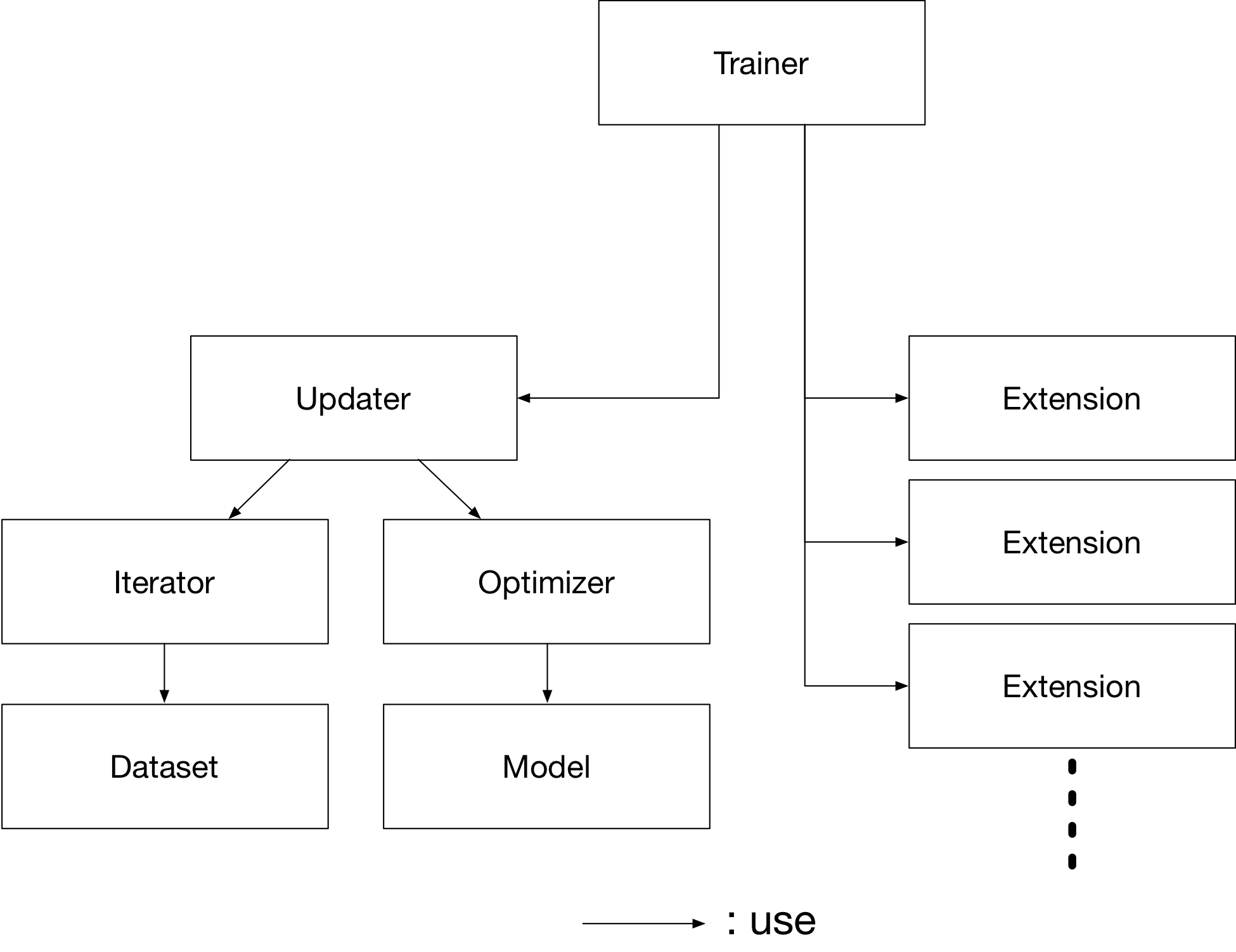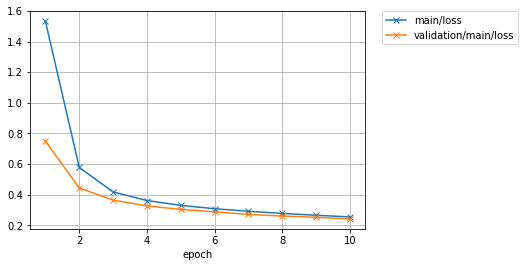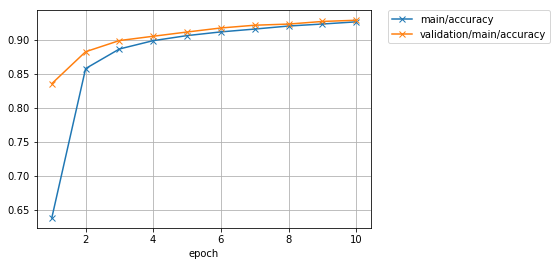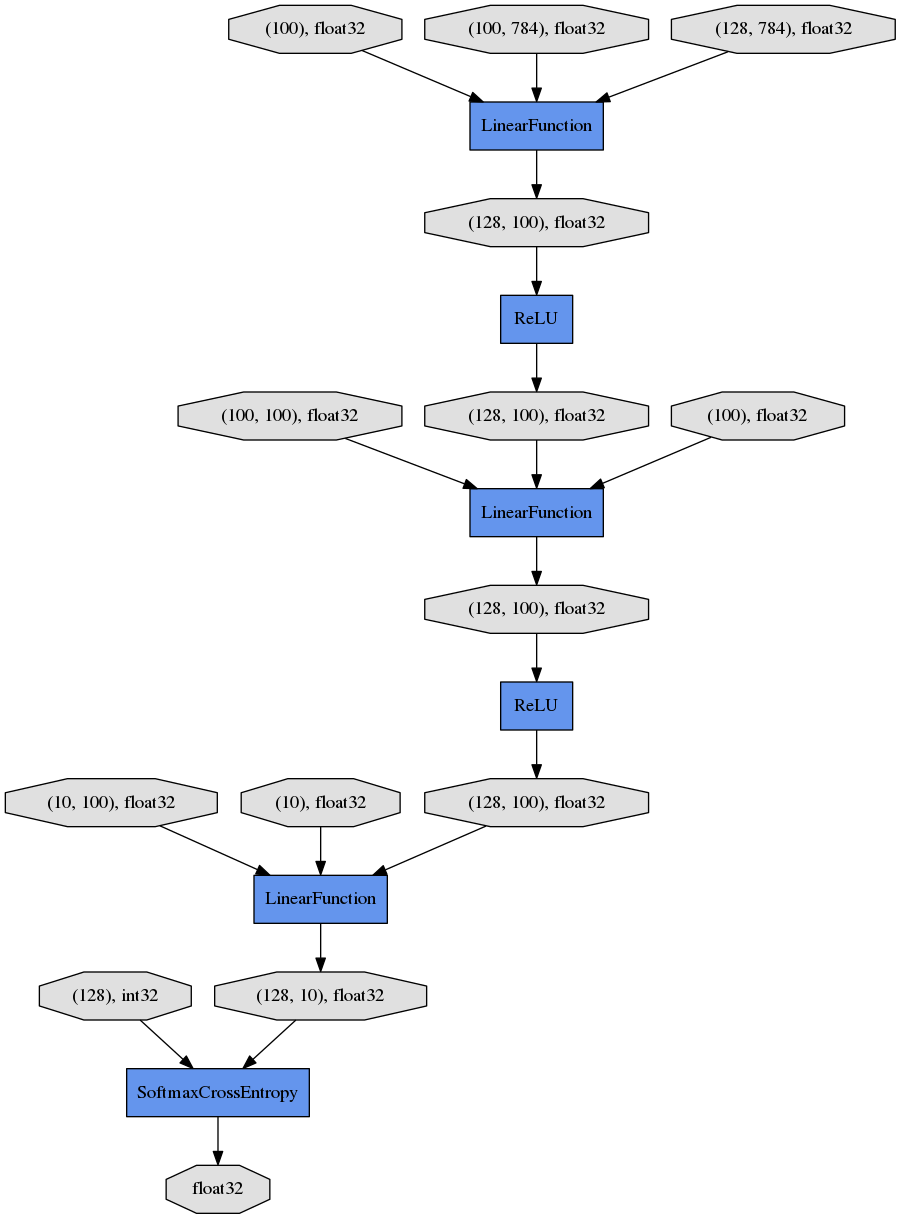# MNIST using Trainer¶

In the example code of this tutorial, we assume for simplicity that the following symbols are already imported.

```import math
import numpy as np
import chainer
from chainer import backend
from chainer import backends
from chainer.backends import cuda
from chainer import Function, FunctionNode, gradient_check, report, training, utils, Variable
from chainer import datasets, initializers, iterators, optimizers, serializers
from chainer import Link, Chain, ChainList
import chainer.functions as F
import chainer.links as L
from chainer.training import extensions
```

By using `Trainer`, you don’t need to write the training loop explicitly any more. Furthermore, Chainer provides many useful extensions that can be used with `Trainer` to visualize your results, evaluate your model, store and manage log files more easily.

This example will show how to use the `Trainer` to train a fully-connected feed-forward neural network on the MNIST dataset.

Note

If you would like to know how to write a training loop without using the `Trainer`, please check MNIST with a Manual Training Loop instead of this tutorial.

## 1. Prepare the dataset¶

Load the MNIST dataset, which contains a training set of images and class labels as well as a corresponding test set.

```from chainer.datasets import mnist

train, test = mnist.get_mnist()
```

Note

You can use a Python list as a dataset. That’s because `Iterator` can take any object as a dataset whose elements can be accessed via `[]` accessor and whose length can be obtained with `len()` function. For example,

```train = [(x1, t1), (x2, t2), ...]
```

a list of tuples like this can be used as a dataset.

There are many utility dataset classes defined in `datasets`. It is recommended that you utilize them in the actual applications.

For example, if your dataset consists of a number of image files, it would take a large amount of memory to load those data into a list like above. In that case, you can use `ImageDataset`, which just keeps the paths to image files. The actual image data will be loaded from the disk when the corresponding element is requested via `[]` accessor. Until then, no images are loaded to the memory to reduce memory use.

## 2. Prepare the dataset iterations¶

`Iterator` creates a mini-batch from the given dataset.

```batchsize = 128

train_iter = iterators.SerialIterator(train, batchsize)
test_iter = iterators.SerialIterator(test, batchsize, False, False)
```

## 3. Prepare the model¶

Here, we are going to use the same model as the one defined in MNIST with a Manual Training Loop.

```class MLP(Chain):

def __init__(self, n_mid_units=100, n_out=10):
super(MLP, self).__init__()
with self.init_scope():
self.l1 = L.Linear(None, n_mid_units)
self.l2 = L.Linear(None, n_mid_units)
self.l3 = L.Linear(None, n_out)

def forward(self, x):
h1 = F.relu(self.l1(x))
h2 = F.relu(self.l2(h1))
return self.l3(h2)

gpu_id = 0  # Set to -1 if you use CPU

model = MLP()
if gpu_id >= 0:
model.to_gpu(gpu_id)
```

## 4. Prepare the Updater¶

`Trainer` is a class that holds all of the necessary components needed for training. The main components are shown below.Basically, all you need to pass to `Trainer` is an `Updater`. However, `Updater` contains an `Iterator` and `Optimizer`. Since `Iterator` can access the dataset and `Optimizer` has references to the model, `Updater` can access to the model to update its parameters.

So, `Updater` can perform the training procedure as shown below:

1. Retrieve the data from dataset and construct a mini-batch (`Iterator`)

2. Pass the mini-batch to the model and calculate the loss

3. Update the parameters of the model (`Optimizer`)

Now let’s create the `Updater` object !

```max_epoch = 10

# Wrap your model by Classifier and include the process of loss calculation within your model.
# Since we do not specify a loss function here, the default 'softmax_cross_entropy' is used.
model = L.Classifier(model)

# selection of your optimizing method
optimizer = optimizers.MomentumSGD()

# Give the optimizer a reference to the model
optimizer.setup(model)

# Get an updater that uses the Iterator and Optimizer
updater = training.updaters.StandardUpdater(train_iter, optimizer, device=gpu_id)
```

Note

Here, the model defined above is passed to `Classifier` and changed to a new `Chain`. `Classifier`, which in fact inherits from the `Chain` class, keeps the given `Chain` model in its `predictor` attribute. Once you give the input data and the corresponding class labels to the model by the `()` operator,

1. `forward()` of the model is invoked. The data is then given to `predictor` to obtain the output `y`.

2. Next, together with the given labels, the output `y` is passed to the loss function which is determined by `lossfun` argument in the constructor of `Classifier`.

3. The loss is returned as a `Variable`.

In `Classifier`, the `lossfun` is set to `softmax_cross_entropy()` as default.

`StandardUpdater` is the simplest class among several updaters. There are also the `ParallelUpdater` and the `MultiprocessParallelUpdater` to utilize multiple GPUs. The `MultiprocessParallelUpdater` uses the NVIDIA NCCL library, so you need to install NCCL and re-install CuPy before using it.

## 5. Setup Trainer¶

Lastly, we will setup `Trainer`. The only requirement for creating a `Trainer` is to pass the `Updater` object that we previously created above. You can also pass a `stop_trigger` to the second trainer argument as a tuple like `(length, unit)` to tell the trainer when to stop the training. The `length` is given as an integer and the `unit` is given as a string which should be either `epoch` or `iteration`. Without setting `stop_trigger`, the training will never be stopped.

```# Setup a Trainer
trainer = training.Trainer(updater, (max_epoch, 'epoch'), out='mnist_result')
```

The `out` argument specifies an output directory used to save the log files, the image files of plots to show the time progress of loss, accuracy, etc. when you use `PlotReport` extension. Next, we will explain how to display or save those information by using trainer `Extension`.

## 6. Add Extensions to the Trainer object¶

The `Trainer` extensions provide the following capabilities:

To use these wide variety of tools for your training task, pass `Extension` objects to the `extend()` method of your `Trainer` object.

```from chainer.training import extensions

trainer.extend(extensions.LogReport())
trainer.extend(extensions.snapshot(filename='snapshot_epoch-{.updater.epoch}'))
trainer.extend(extensions.snapshot_object(model.predictor, filename='model_epoch-{.updater.epoch}'))
trainer.extend(extensions.Evaluator(test_iter, model, device=gpu_id))
trainer.extend(extensions.PrintReport(['epoch', 'main/loss', 'main/accuracy', 'validation/main/loss', 'validation/main/accuracy', 'elapsed_time']))
trainer.extend(extensions.PlotReport(['main/loss', 'validation/main/loss'], x_key='epoch', file_name='loss.png'))
trainer.extend(extensions.PlotReport(['main/accuracy', 'validation/main/accuracy'], x_key='epoch', file_name='accuracy.png'))
trainer.extend(extensions.DumpGraph('main/loss'))
```

### `LogReport`¶

Collect `loss` and `accuracy` automatically every `epoch` or `iteration` and store the information under the `log` file in the directory specified by the `out` argument when you create a `Trainer` object.

### `snapshot()`¶

The `snapshot()` method saves the `Trainer` object at the designated timing (default: every epoch) in the directory specified by `out`. The `Trainer` object, as mentioned before, has an `Updater` which contains an `Optimizer` and a model inside. Therefore, as long as you have the snapshot file, you can use it to come back to the training or make inferences using the previously trained model later.

### `snapshot_object()`¶

However, when you keep the whole `Trainer` object, in some cases, it is very tedious to retrieve only the inside of the model. By using `snapshot_object()`, you can save the particular object (in this case, the model wrapped by `Classifier`) as a separate snapshot. `Classifier` is a `Chain` object which keeps the model that is also a `Chain` object as its `predictor` property, and all the parameters are under the `predictor`, so taking the snapshot of `predictor` is enough to keep all the trained parameters.

This is a list of commonly used trainer extensions:

`LogReport`

This extension collects the loss and accuracy values every epoch or iteration and stores in a log file. The log file will be located under the output directory (specified by `out` argument of the `Trainer` object).

`snapshot()`

This extension saves the `Trainer` object at the designated timing (defaut: every epoch) in the output directory. The `Trainer` object, as mentioned before, has an `Updater` which contains an `Optimizer` and a model inside. Therefore, as long as you have the snapshot file, you can use it to come back to the training or make inferences using the previously trained model later.

`snapshot_object()`

`snapshot()` extension above saves the whole `Trainer` object. However, in some cases, it is tedious to retrieve only the inside of the model. By using `snapshot_object()`, you can save the particular object (in the example above, the model wrapped by `Classifier`) as a separeted snapshot. Taking the snapshot of `predictor` is enough to keep all the trained parameters, because `Classifier` (which is a subclass of `Chain`) keeps the model as its `predictor` property, and all the parameters are under this property.

`DumpGraph()`

This extension saves the structure of the computational graph of the model. The graph is saved in Graphviz dot format under the output directory of the `Trainer`.

`Evaluator`

`Iterator`s that use the evaluation dataset and the model object are required to use `Evaluator` extension. It evaluates the model using the given dataset (typically it’s a validation dataset) at the specified timing interval.

`PrintReport`

This extension outputs the spcified values to the standard output.

`PlotReport`

This extension plots the values specified by its arguments and saves it as a image file.

This is not an exhaustive list of built-in extensions. Please take a look at Extensions for more of them.

## 7. Start Training¶

Just call `run()` method from `Trainer` object to start training.

```trainer.run()
```
```epoch       main/loss   main/accuracy  validation/main/loss  validation/main/accuracy  elapsed_time
1           1.53241     0.638409       0.74935               0.835839                  4.93409
2           0.578334    0.858059       0.444722              0.882812                  7.72883
3           0.418569    0.886844       0.364943              0.899229                  10.4229
4           0.362342    0.899089       0.327569              0.905558                  13.148
5           0.331067    0.906517       0.304399              0.911788                  15.846
6           0.309019    0.911964       0.288295              0.917722                  18.5395
7           0.292312    0.916128       0.272073              0.921776                  21.2173
8           0.278291    0.92059        0.261351              0.923457                  23.9211
9           0.266266    0.923541       0.253195              0.927314                  26.6612
10          0.255489    0.926739       0.242415              0.929094                  29.466
```

Let’s see the plot of loss progress saved in the `mnist_result` directory.How about the accuracy?Furthermore, let’s visualize the computational graph saved with `DumpGraph()` using Graphviz.

```% dot -Tpng mnist_result/cg.dot -o mnist_result/cg.png
```From the top to the bottom, you can see the data flow in the computational graph. It basically shows how data and parameters are passed to the `Function`s.

## 8. Evaluate a pre-trained model¶

Evaluation using the snapshot of a model is as easy as what explained in the MNIST with a Manual Training Loop.

```import matplotlib.pyplot as plt

model = MLP()

# Show the output
x, t = test
plt.imshow(x.reshape(28, 28), cmap='gray')
plt.show()
print('label:', t)

y = model(x[None, ...])

print('predicted_label:', y.array.argmax(axis=1))
``````label: 7
predicted_label: 7
```

The prediction looks correct. Success!